# Video Library

Included in this video library are a series of video clips that have been carefully vetted and selected by staff from the Center for Advanced Study of Teaching and Learning (CASTL). Video clips on specific topics can be narrowed down using the categories on the left.

## 53 videos currently available

Showing results: 1 through 16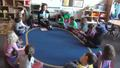skill: Adding and Subtracting strategy: Supporting conceptual understanding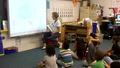skill: Adding and Subtracting strategy: Asking children open-ended questions that elicit their solution-methods strategy: Extending mathematical thinking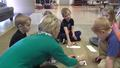skill: Adding and Subtracting strategy: Extending mathematical thinking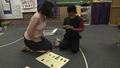Adding Dots Together on a DominoBuilding 3-D Shapes with Magnet Shapes

skill: Shape Recognition and Properties strategy: Modeling and using math language strategy: Supporting conceptual understanding strategy: Extending mathematical thinking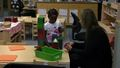Building a Tower with Shapes

skill: Shape Recognition and Properties strategy: Modeling and using math language strategy: Extending mathematical thinking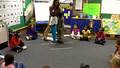Comparing Children's Height

skill: Describing and Comparing strategy: Modeling and using math language strategy: Asking children open-ended questions that elicit their solution-methods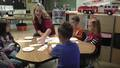Comparing Groups of Gold Bars

skill: Comparing and Ordering strategy: Modeling and using math language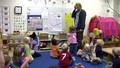Comparing Numbers While Tracking the Weather

skill: Comparing and Ordering strategy: Modeling and using math language strategy: Asking children open-ended questions that elicit their solution-methods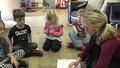Comparing the Appearance of Numerals

skill: Recognizing and Writing Numerals strategy: Modeling and using math language strategy: Asking children open-ended questions that elicit their solution-methods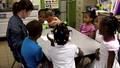Comparing the Volume of Containers Using Rice

skill: Describing and Comparing strategy: Modeling and using math language strategy: Extending mathematical thinking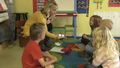Comparing the Weight of a Golf Ball and a Ping Pong Ball

skill: Describing and Comparing strategy: Modeling and using math language strategy: Asking children open-ended questions that elicit their solution-methods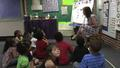skill: Comparing and Ordering strategy: Modeling and using math language strategy: Asking children open-ended questions that elicit their solution-methods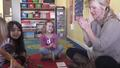Counting and Producing a Set of Pennies for Shopping

skill: Counting and Cardinality strategy: Modeling and using math language strategy: Supporting conceptual understanding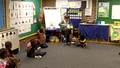Counting Frogs During Storytime

skill: Counting and Cardinality strategy: Supporting conceptual understanding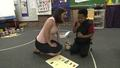Counting On to Determine How Many Dots

skill: Adding and Subtracting strategy: Supporting conceptual understanding
Showing results: 1 through 16